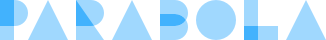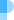HomeFeaturesExamplesPricingLearn

# Calculating running total

Calculate a running total of values within a column of a table### Objective

Calculating a running total is useful for many types of reporting, but it remains a manual process, which is inflexible to new data is introduced. In Excel, you can use a custom SUM formula, but if you add new rows, you will need to recopy the formula into the new cells. In SQL you can use a self join or a window function that sums previous rows.

### Solution

Using the Running Total Object, you can automatically append a running total to any table. In this example, we take a table with many columns that need to contribute to the running total and use the Unpivot Object to line up all of the columns that need to be summed. Then, using the Group By Object, we sum all of the values per email. From this data, we can construct the running total.